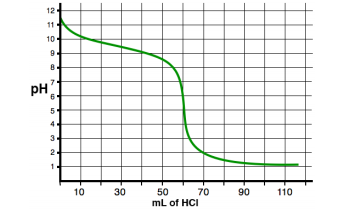# Problem: Below is the pH curve for a titration of a weak base with 0.10 M HCl. What is the p  Kb of the weak base? 1. 9.42. 2.73. 4.64. 7.00

###### FREE Expert Solution
93% (449 ratings)
###### Problem Details

Below is the pH curve for a titration of a weak base with 0.10 M HCl. What is the p  Kb of the weak base?

1. 9.4

2. 2.7

3. 4.6

4. 7.00Frequently Asked Questions

What scientific concept do you need to know in order to solve this problem?

Our tutors have indicated that to solve this problem you will need to apply the Acid and Base Titration Curves concept. You can view video lessons to learn Acid and Base Titration Curves. Or if you need more Acid and Base Titration Curves practice, you can also practice Acid and Base Titration Curves practice problems.

What is the difficulty of this problem?

Our tutors rated the difficulty ofBelow is the pH curve for a titration of a weak base with 0....as medium difficulty.

How long does this problem take to solve?

Our expert Chemistry tutor, Jules took 4 minutes and 7 seconds to solve this problem. You can follow their steps in the video explanation above.

What professor is this problem relevant for?

Based on our data, we think this problem is relevant for Professor Old's class at UCB.